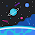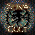## 2012-05-28

### #11 - libgdx Tutorial: Vectors

Libgdx version used on this post: 0.9.3 (download)
Hi guys! I've been extremely busy with some parallel projects, but as I received some donations and amazing feedbacks as well, I'll find some time to write more posts! In this one we'll talk about vectors, an element that allows us to represent positions, speeds, velocities, accelerations, directions, distances and so on.

## Important changes

• Updated the version of libgdx to the latest version available. All I had to do was to download the latest build and replace the JARs and .so files in our projects.

## Why should we use vectors?

Vectors are cool because they can represent some informations in a way that's easy to understand, handle and combine. Basically they represent a direction or a point within a coordinate system, optionally with an associated scalar value.

So far we've dealt only with velocity, but we also want to add acceleration so that the ship's movement seems more real. Using vectors we can easily calculate the final position of the ship after both forces were applied just by combining them! If you want to study the vector concept in depth you can check out this Wikipedia page.

With a vector in hands we can do the following operations:
• Add/subtract other vector to/from it;
• Multiply it by a scalar value;
• Calculate its length, which gives us the associated scalar value;
• Normalize it, which makes its length be 1;
• Calculate its angle relative to the x-axis;
• Rotate it by a given angle;
• Calculate its distance to another vector.

Libgdx provides two vector implementations:

As you can see, they belong to the com.badlogic.gdx.math package, which is very useful. It provides implementations of things like circles, planes, polygons, rectangles, spheres and so on. I suggest you take some time to study this package in details. Maybe one day I'll write about it.

## Using a position vector

As an example, we will change our game to use a vector to represent the ship's position. Let's start by modifying the Ship2D class to include the following vector attribute:
```    private Vector2 position; // unitless
```
Sadly the (x,y) position coordinates of an actor are not wrapped into a Vector2 object. That's why we're using a position vector. At specific moments we'll have to update the actor's position based on our position vector. Now we could rewrite our Ship2D#moveShip method as follows (I omitted some code in favor of readability):
```    private void moveShip(
float delta )
{
// set the position vector to the ship's current position
position.set( x, y );

// move UP or DOWN
if( Gdx.input.isKeyPressed( Input.Keys.UP ) ) {
position.add( 0, MAX_VERTICAL_SPEED * delta );
} else if( Gdx.input.isKeyPressed( Input.Keys.DOWN ) ) {
position.sub( 0, MAX_VERTICAL_SPEED * delta );
}

// move LEFT or RIGHT
if( Gdx.input.isKeyPressed( Input.Keys.LEFT ) ) {
position.sub( MAX_HORIZONTAL_SPEED * delta, 0 );
} else if( Gdx.input.isKeyPressed( Input.Keys.RIGHT ) ) {
position.add( MAX_HORIZONTAL_SPEED * delta, 0 );
}

// update the ship's actual position
x = position.x;
y = position.y;
}
```

## Using velocity and acceleration vectors

We're using vectors now, but the result was exactly the same as before! When we add the acceleration vector we'll see all the magic happen. First, let's understand the difference between speed, velocity and acceleration:
• Speed is a scalar quantity that tells us how fast an object is moving; eg: 10m/s.
• Velocity is a vector quantity that tells us how fast an object is moving and in what direction; eg: 10m/s east.
• Acceleration is a vector quantity that tells us the rate of change of velocity; eg: 10m/s² south.
So what we need to do now is:
1. Calculate the acceleration based on the user input.
2. Modify the ship's velocity based on the calculated acceleration.
3. Update the ship's position based on the modified velocity.
And we should enforce some rules:
• The maximum acceleration will be 8px/s². When using the keyboard, this maximum value is reached immediately, but when using the device's accelerator it should be calculated.
• The maximum speeds (horizontal and vertical) must be respected.
• When reaching the boundaries, the ship's velocity should be zeroed, so that the ship stops flying in the current direction.

## Modifying the Ship2D#moveShip method

We need to set the maximum acceleration, just like we set the maximum speed:
```    private static final float MAX_ACCELERATION = 8; // unit: px/s²
```
And we need the new vectors:
```    private Vector2 velocity; // unit: px/s
private Vector2 acceleration; // unit: px/s²
```
Now, let's follow our plan:

1) Calculate the acceleration based on the user input
The acceleration should be set as follows:
• User is pressing the left arrow key: acceleration.x = - MAX_ACCELERATION
• User is pressing the right arrow key: acceleration.x = MAX_ACCELERATION
• User is pressing the up arrow key: acceleration.y = MAX_ACCELERATION
• User is pressing the down arrow key: acceleration.y = - MAX_ACCELERATION
We must not forget to adjust the value based on the current delta!
• acceleration = acceleration * delta

2) Modify the ship's velocity based on the calculated acceleration
That's easy:
• velocity = velocity + acceleration
• check the max speed

3) Update the ship's position based on the modified velocity
That's easy too now that we have vectors:
• position = position + velocity
• check the boundaries

## The result

Once again, I'll just detail the keyboard input here because it's simpler. Later you can check out the full source code for the Ship2D class with detailed inline comments.
```    private void moveShip( float delta )
{
// calculate the horizontal and vertical acceleration
acceleration.x = ( Gdx.input.isKeyPressed( Input.Keys.LEFT ) ? - MAX_ACCELERATION
: ( Gdx.input.isKeyPressed( Input.Keys.RIGHT ) ? MAX_ACCELERATION : 0 ) );
acceleration.y = ( Gdx.input.isKeyPressed( Input.Keys.UP ) ? MAX_ACCELERATION
: ( Gdx.input.isKeyPressed( Input.Keys.DOWN ) ? - MAX_ACCELERATION : 0 ) );

// note that when the keys are not pressed, the acceleration will be
// zero, so the ship's velocity won't be affected

// apply the delta on the acceleration
acceleration.mul( delta );

// modify the ship's velocity

// check the max speed
if( velocity.x < 0 ) {
velocity.x = Math.max( velocity.x, - MAX_HORIZONTAL_SPEED * delta );
} else if( velocity.x > 0 ) {
velocity.x = Math.min( velocity.x, MAX_HORIZONTAL_SPEED * delta );
}
if( velocity.y < 0 ) {
velocity.y = Math.max( velocity.y, - MAX_VERTICAL_SPEED * delta );
} else if( velocity.y > 0 ) {
velocity.y = Math.min( velocity.y, MAX_VERTICAL_SPEED * delta );
}

// update the ship's position

// make sure the ship is inside the stage
if( position.x < 0 ) {
position.x = 0;
velocity.x = 0;
} else if( position.x > stage.width() - width ) {
position.x = stage.width() - width;
velocity.x = 0;
}
if( position.y < 0 ) {
position.y = 0;
velocity.y = 0;
} else if( position.y > stage.height() - height ) {
position.y = stage.height() - height;
velocity.y = 0;
}

// update the ship's actual position
x = position.x;
y = position.y;
}
```

## Conclusion

We have implemented acceleration and the ship's behaviour seems more real! Features like these are absolutely necessary if we want the player to be able to immerse in the game. In the next post the ship will tilt to the side its moving, and then we'll move on to another actors!

Here is the Subversion repository tag for this post. Thanks!

1.Thanks, I was hoping you didn't forget us... ;)

Quick question: Maybe I got something wrong, but with the svn code of #10 my ship is already tilting when using the phone.

2.Hi Simon! Don't worry! :)
I'm still motivated to write this tutorial!

What I mean with tilt is that when moving the ship horizontaly, it should incline to the side it's moving. And if you check out the image atlas, we already have the images for that!

http://3.bp.blogspot.com/-S-OG4MfE-DQ/T2nAjvLx97I/AAAAAAAABAM/1KzOaKQfasI/s1600/tyrian.shp.007D3C.png

Thanks for your support!

1.Thanks for the answer. I guess, I'm getting old. I could swear the ship was already inclining on my phone. Making some screenshots while moving it prooved me and my crappy eyes wrong...

Sorry for the unnecessary comment and keep up your awesome work! :)

2.That's no problem at all! :)

3.Excellent stuff!

1.Thanks! I noticed my blog was listed in the "external tutorials" section of the new libgdx web-site!

That's awesome! :)

4.Thanks for step by stem instructions. We are waiting for new chapter :)

1.I've already commited the code for the next post, but I need to find the time to write it. There is a chance I find some time in the weekend!

5.Hi Gustavo, i saw the comment you did in http://ericharlow.blogspot.com.br/2010/09/experience-multiple-android-activities.html?showComment=1316094935403#c2968554094913314597.

I have the same problem, did you solve it?

Thank you!

1.Hi Juliano! Sadly I couldn't fix it, so I ended up changing my application's screen flow.
Sorry I couldn't help!

6.Fantastic work! I'm using this to set up a skeleton app to use in all of my (hopefully) coming projects. 3 beers headed your way!

1.Good luck with your projects, and thanks for your donation!
And yeah, I plan to finish the game and even submit it to the market. :)

7.Hi Gustavo,

Thank you very much for this tutorial. ;)

Maybe I did not notice where you explained it, but I do not
understand how you do to calculate MAX_ACCELERATION.

Why it should be 8 px/s² ?

8.Hi gustavo I followed your tutorial and based a project of my own on it, could you give me some pointers on how to add the bullets and enemies. Should I use the levelscreen to create all these ?? My plan was to spawn time based enemy/bullet patterns and load these and the background based on the levelId. My code is here:https://github.com/silconsystem/Gensokyo_LibGdx.git

Great tutorial, I learned a lot from you thank you !

9.THX A LOT
with ur tutorials, i can make my JUKEBOX-PRINT-N-SING